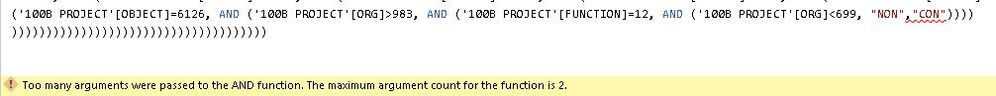cancel
Showing results for
Did you mean:

Fabric is Generally Available. Browse Fabric Presentations. Work towards your Fabric certification with the Cloud Skills Challenge.Anonymous
Not applicable

## DAX FUNCTION

I have a formula from excel and it really works and produces the output needed. However I need to convert this formula into Dax and use it in PowerBI. I have tried both || and && and its still not working: Here is the excel formula==>

=IF(OR(AND(OBJECT="6265",VALUE(ORG)<“699”),OBJECT="6255",AND(OBJECT="6256",VALUE(ORG)<“699”),OBJECT="6257",OBJECT="6258",OBJECT="6259",OBJECT="8911",AND(OBJECT="6112",VALUE(ORG)<“699”),AND(SUB_OBJECT="XH",VALUE(ORG)<>“816”),SUB_OBJECT="LQ",AND(SUB_OBJECT="DF",VALUE(ORG)<>”961”),AND(SUB_OBJECT="TE",VALUE(ORG)<>“816”),AND(SUB_OBJECT="JF",VALUE(ORG)<“699”),AND(SUB_OBJECT="JP",VALUE(ORG)<“699”),AND(SUB_OBJECT="CM",VALUE(ORG)<>“816”),AND(SUB_OBJECT="48",VALUE(ORG)<>“969”),OBJECT="6116",OBJECT="6119",OBJECT="6129",OBJECT="6141",OBJECT="6142",OBJECT="6143",OBJECT="6145",OBJECT="6146",OBJECT="6149",OBJECT="6144",OBJECT="6113",OBJECT="6126",VALUE(ORG)>“983”,AND(FUNCTION="12",VALUE(ORG)<“699”)),"NON","CON")

Note - All the the numbers here are text formats.

Thanks.

2 ACCEPTED SOLUTIONSMicrosoft

Hi @Anonymous,

You use the VALUE function, which converts a text string that represents a number to a number. For example, VALUE("3") returns number 3.  What's the type of ORG column? It is text like "32", then VALUE(ORG) returns number type, which is nou used to compare with text type, like VALUE(ORG)<“699”. Mybe you can change it to VALUE(ORG)<VALUE(“699”), and also mix corresponding arguments in @KHorseman posted formula using similar way. Please try and check if it work fine.

Best Regards,
AngeliaCommunity Champion

You need to indent and format your code so you can read it. You have the wrong number of parentheses in the wrong places.

Proud to be a Super User!

12 REPLIES 12Community Champion

Is this a column or a measure? Is Object a column? Org? Function? What exactly do you mean "it's not working"? What output are you getting? What output are you expecting?

Proud to be a Super User!Anonymous
Not applicable

Sorry these are all columns (object, org, function, sub_object). I m trying to create a now column from that formula. It create a new column in excel perfectly but not in PowerBI or DAXCommunity Champion

Your first OR function seems to have about a dozen arguments when it is supposed to only have 2, so I assume you're just getting an error message?

Proud to be a Super User!Community Champion

Actually, it has 30 arguments. I've formatted your code so it's readable.

```=IF(
OR(
AND(
OBJECT="6265",
VALUE(ORG)<“699”
),
OBJECT="6255",
AND(
OBJECT="6256",
VALUE(ORG)<“699”
),
OBJECT="6257",
OBJECT="6258",
OBJECT="6259",
OBJECT="8911",
AND(
OBJECT="6112",
VALUE(ORG)<“699”
),
AND(
SUB_OBJECT="XH",
VALUE(ORG)<>“816”
),
SUB_OBJECT="LQ",
AND(
SUB_OBJECT="DF",
VALUE(ORG)<>”961”
),
AND(
SUB_OBJECT="TE",
VALUE(ORG)<>“816”
),
AND(
SUB_OBJECT="JF",
VALUE(ORG)<“699”
),
AND(
SUB_OBJECT="JP",
VALUE(ORG)<“699”
),
AND(
SUB_OBJECT="CM",
VALUE(ORG)<>“816”
),
AND(
SUB_OBJECT="48",
VALUE(ORG)<>“969”
),
OBJECT="6116",
OBJECT="6119",
OBJECT="6129",
OBJECT="6141",
OBJECT="6142",
OBJECT="6143",
OBJECT="6145",
OBJECT="6146",
OBJECT="6149",
OBJECT="6144",
OBJECT="6113",
OBJECT="6126",
VALUE(ORG)>“983”,
AND(
FUNCTION="12",
VALUE(ORG)<“699”
)
),
"NON",
"CON"
)```

Columns should be referred to in square brackets, by the way. TableName[Object] for instance.

Proud to be a Super User!Anonymous
Not applicable

I refered the columns by their name instance and still got the error mesage that too many arguments were passed to the VALUE function. The maximun argument count for the function is 1.Community Champion

You need to indent and format your code so you can read it. You have the wrong number of parentheses in the wrong places.

Proud to be a Super User!Microsoft

Hi @Anonymous,

You use the VALUE function, which converts a text string that represents a number to a number. For example, VALUE("3") returns number 3.  What's the type of ORG column? It is text like "32", then VALUE(ORG) returns number type, which is nou used to compare with text type, like VALUE(ORG)<“699”. Mybe you can change it to VALUE(ORG)<VALUE(“699”), and also mix corresponding arguments in @KHorseman posted formula using similar way. Please try and check if it work fine.

Best Regards,
AngeliaCommunity Champion

You need to be specific about what you mean by "not working". Are you getting an error message? What error message?

Proud to be a Super User!Community Champion

```=IF(
AND(
TableName[OBJECT] = "6265",
VALUE(TableName[ORG])<“699”
) ||
TableName[OBJECT]="6255" ||
AND(
TableName[OBJECT]="6256",
VALUE(TableName[ORG])<“699”
) ||

.... etc etc ```

Proud to be a Super User!Anonymous
Not applicable

Here is the error message:Anonymous
Not applicable

The error message was about the number of acceptable arguments- saying it only accepts 2Anonymous
Not applicable

Yes I get that error message but what if I want to use multiple arguments outside of excel? I read from another forum about combining  arguments with either && or || but its still not working.Announcements#### Power BI Monthly Update - November 2023

Check out the November 2023 Power BI update to learn about new features.#### Fabric Community News unified experience

Read the latest Fabric Community announcements, including updates on Power BI, Synapse, Data Factory and Data Activator.#### The largest Power BI and Fabric virtual conference

130+ sessions, 130+ speakers, Product managers, MVPs, and experts. All about Power BI and Fabric. Attend online or watch the recordings.Top Solution Authors
Top Kudoed Authors
Users online (4,036)